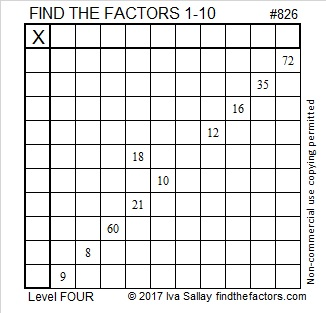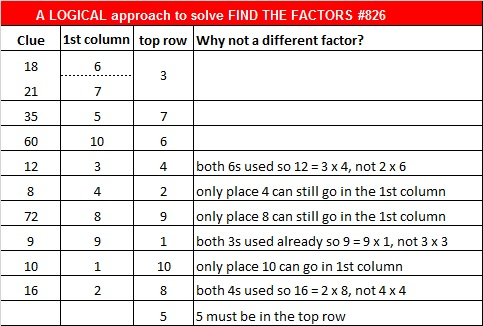How to Find Consecutive Numbers That Sum to 826

Most numbers greater than 2 can be written as the sum of consecutive numbers. How can you know what those consecutive numbers are? By factoring, of course! Let’s take 826 as an example.

To find consecutive numbers that add up to 826, we are only interested in its odd factors that are less than or equal to 40 AND its factor that is the greatest power of 2. (We arrived at the number 40 because the largest triangular number less than 826 is the 40th triangular number, 820. We could also find the number 40 if we round √(1 + 826×2) – 1 to the nearest whole number.)

The factor of 820 that is the greatest power of 2 is 2. When we double that greatest power of 2, we get 4. The odd factors of 826 that are less than or equal to 40 are 1 and 7. Now we don’t ever count a number being the sum of just 1 consecutive number. So for 826, we are interested in just three numbers, all of which are less than or equal to the maximum number allowable, 40. Those numbers are 7, 1×4, and 7×4.

Thus, we can conclude that 826 can be written as the sum of 4 consecutive numbers, 7 consecutive numbers, and 28 consecutive numbers. Can you figure out what all those consecutive numbers are? How are the consecutive number sums derived from an odd factor different from the sums derived from an even number?

I’ve written out the sum of 4 consecutive numbers as an example and given some hints to help you figure out or check your answer to those two questions:

• 826÷4 = 206.5, and that number lies right smack in the middle of the 4 consecutive numbers that make this sum: 205 + 206 + 207 + 208 = 826. Note that 205 + 208 and 206 + 207 both add up to 413, a factor of 826.
• 826÷7 = 118, which is the exact middle number of the 7 consecutive numbers that sum up to 826. Note that 7 × 118 = 826.
• 826÷28 = 29.5 so the 14 numbers from 16 to 29 plus the 14 numbers from 30 to 43 make the 28 numbers from 16 to 43 that add up to 826. Note that 16 + 43 = 59, a factor of 826.

Here’s today’s puzzle:Print the puzzles or type the solution on this excel file: 10-factors-822-828

In order for (821, 823, 827, 829) to be the 4th prime decade, 825 or one of the even numbers between 820 and 830 has to be divisible by 7.

826 is the one that answered that call. Here is the 7 divisibility trick applied to 826:

• 82-2(6) = 70, a number divisible by 7, so 826 is divisible by 7.

Why does 826 become the palindrome 181 in BASE 25? Because 1(25²) + 8(25¹) + 1(25º) = 826

Let’s begin with 826’s factoring information:

• 826 is a composite number.
• Prime factorization: 826 = 2 × 7 × 59
• The exponents in the prime factorization are 1, 1, and 1. Adding one to each and multiplying we get (1 + 1)(1 + 1)(1 + 1) = 2 × 2 × 2 = 8. Therefore 826 has exactly 8 factors.
• Factors of 826: 1, 2, 7, 14, 59, 118, 413, 826
• Factor pairs: 826 = 1 × 826, 2 × 413, 7 × 118, or 14 × 59
• 826 has no square factors that allow its square root to be simplified. √826 ≈ 28.7402